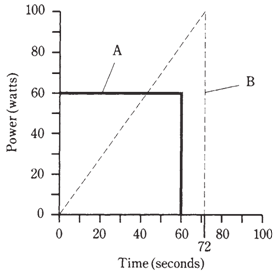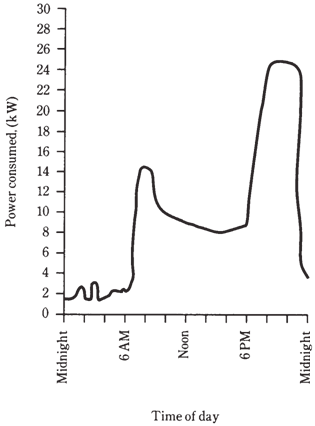## Energy Assignment Help

Assignment Help: >> Electrical Engineering - Energy

Energy and the watt hour

There is difference in between energy and power. You have probably heard the 2 terms used interchangeably, as if they mean same thing, however they do not Energy is power dissipated over the length of time. Power is rate at which energy can be expended.

The unit of energy or we can say that energy can be measured in in joules. One joule is the equivalent of 1 watt of power, dissipated for 1 second of time. In electricity, you will often encounter watt hour or kilowatt hour. As their names signify, a watt hour, abbreviated Wh, is the equivalent of 1 W dissipated for an hour, and 1 kilowatt hour is the equivalent of 1 kW of power dissipated for 1 h.

Energy of one Wh can be dissipated in the infinite number of different ways. A 60-watt bulb will burn sixty Wh in an hour, or 1 Wh per minute. A hundred W bulb would burn 1 Wh in 1/100 hour, or 36 seconds. A 6-watt Christmas tree bulb would require 10 min- utes (1/6 hour) to burn 1 Wh. And rate of power dissipation is not required be constant; it could be changing constantly.

Figure given below illustrates 2 hypothetical devices which burn up 1 Wh of energy. Device A uses its power at a constant rate of sixty watts, so it consumes one Wh in a minute. The power consumption rate of device B varies, beginning from zero and ending up at quite a lot more than 60 W. How do you know that this 2nd device burns up 1 Wh of energy? Determine the area under graph. This instance has been chosen because figuring out this area is easy rather. Keep in mind that the area of a triangle is equal to half the product of the base length and the height. This second device is on for seventy two seconds, or 1.2 minute; this is 1.2/60   0.02 hour. Then the area under the "curve" is 1/2*100*0.02=1 Wh.Figure: Two devices that burn 1 Wh of energy. Device A dissipates a constant power; device B dissipates a changing amount of power.

While calculating energy values, you should always remember the units you are using. In this case the unit is watt hour, so you should multiply watts by hours. If you multiply watts by minutes, or watts by seconds, you will get the wrong kind of units in the answer. Which means wrong answer.

At times, the curves in graphs like these are complicated. Consider the graph of power consumption in your home, vs time, for the whole day. It may look like the curve in Figure given to us. Finding the area under this curve is not at all a easy task, if you have only this graph to go by. But there is another method to determine the total energy burned by your household in specific time. That is by the help of electric meter. The electrical energy can be measured in kilowatt hours.Figure--   Hypothetical graph showing the power consumed by a typical household, as a function of the time of day.